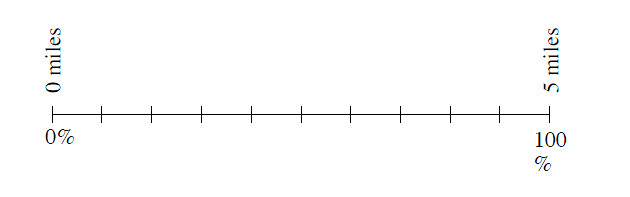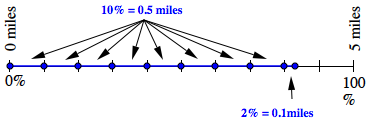### Home > MC1 > Chapter 9 > Lesson 9.1.1 > Problem9-6

9-6.

Alan runs in a race that is $5$ miles long. Copy the percent ruler below and use it to help determine how many miles he has completed after $72\%$ of the race.$10\%$ of $5$ miles is $0.5$ miles. $1\%$ of $5$ miles is $0.05$ miles. Can you use this information to find $82\%$ of $5$ miles?

$3.6$ miles